# Calculating the area and the perimeter

##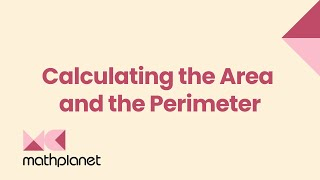By MathPlanetVideos

Calculate the perimeter and the area in feet# Area and Perimeter - Khan Academy

##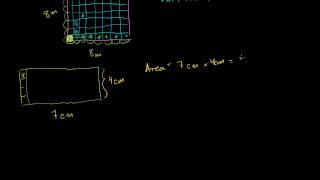By Khan Academy

Start by learning how to calculate the perimeter and area of squares, rectangles, and triangles. Note the explanation of why perimeter measurements are in meters and area measurements are in square meters.# Perimeter and area: the basics | Perimeter, area, and volume | Geometry | Khan Academy

##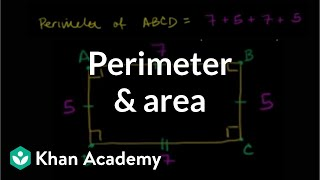By Khan Academy

perimeter and area.# Interesting perimeter and area problems | Perimeter, area, and volume | Geometry | Khan Academy

##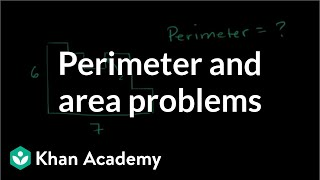By Khan Academy

problems involving perimeter and area# Introduction to perimeter

##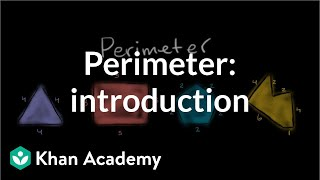By Khan Academy

Find perimeter by adding side-lengths of various figures.# Grade 3 Math Perimeter 3 MD 8

##By EngageNY

Solve real world problems involving perimeters of polygons, including finding the perimeter given the side lengths and finding an unknown side length# Word Problem Involving Perimeter of a Triangle - ex 2

##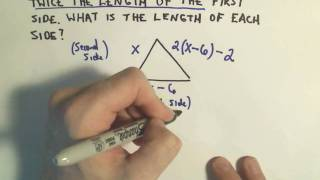By PatrickJMT

YouTube presents Word Problem Involving Perimeter of a Triangle - ex 2, an educational video resource on math.# Word Problem Involving the Perimeter of a Rectangle – Ex 1

##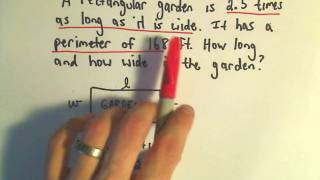By PatrickJMT

YouTube presents Word Problem Involving the Perimeter of a Rectangle, an educational video resource on math.# [4.MD.3-2.0] Area and Perimeter - Common Core Standard

##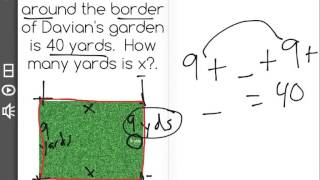By Freckle education

Apply the perimeter formula for rectangles in real world and mathematical problems# Basics of Area Perimeter and Volume of Rectangular Shapes.

##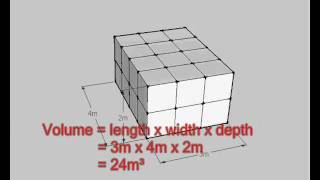By tecmath

This is a basic lesson in working out the perimeter area or volume or rectangular shapes. A house plan is used to show how to find the perimeter of a rectangle. Examples are shown for formulas of perimeter and area.# Area and perimeter problems - rectangular shapes - 3rd/4th grade math

##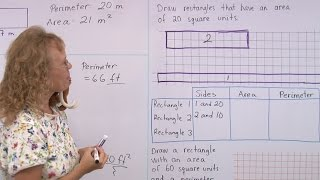By Math Mammoth

After reminding everyone about what is AREA and what is PERIMETER, we solve several problems involving both area and perimeter of rectangles or rectangular shapes.# Perimeter of a shape

##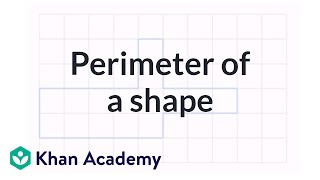By Khan Academy

Find perimeter of various shapes.ï¿½ï¿½ï¿½ï¿½ï¿½ï¿½ï¿½ï¿½ï¿½ï¿½ï¿½ï¿½# Video for Lesson 26: Introduction to Area and Perimeter

##By mathwithlarry

Introductory lesson on area and perimeter. Larry shows examples with a rectangle drawing. More lesson at :http://www.MathWithLarry.com# Perimeter | MathHelp.com

##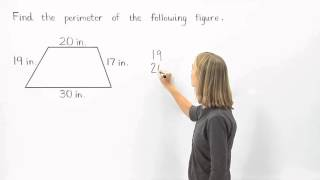By MathHelp.com

This lesson covers naming angles. Students learn the definition of an angle and the concept of measure, including the following vocabulary: side, vertex, protractor, degrees, right angle, acute angle, obtuse angle, and straight angle. Students also learn what assumptions can and cannot be made from a given figure.# Finding perimeter when a side length is missing | Math | 3rd grade | Khan Academy

##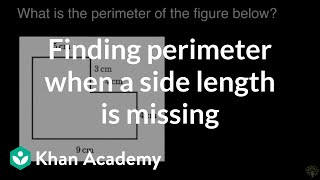By Khan Academy

Lindsay finds the perimeter of a figure when a side length is not given.# Perimeter 2

##By Khan Academy

Find perimeter by adding side-lengths of various figures.# Finding perimeter

##By Khan Academy

Find perimeter by adding side-lengths of various figures.# Finding perimeter

##By Khan Academy

Find perimeter of various shapes.ï¿½ï¿½ï¿½ï¿½ï¿½ï¿½ï¿½ï¿½ï¿½ï¿½ï¿½ï¿½# Math Antics - Perimeter

##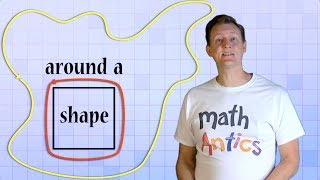By mathantics# Cylinder volume and surface area | Perimeter, area, and volume | Geometry | Khan Academy

##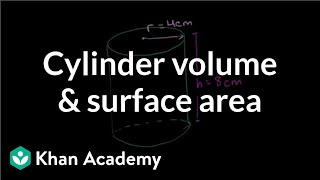By Khan Academy

The other frequently occurring shape is the cylinder. This video will introduce the cylinder and teach you the formulas for finding its surface area and volume.